# Exponential & Trigonometry notes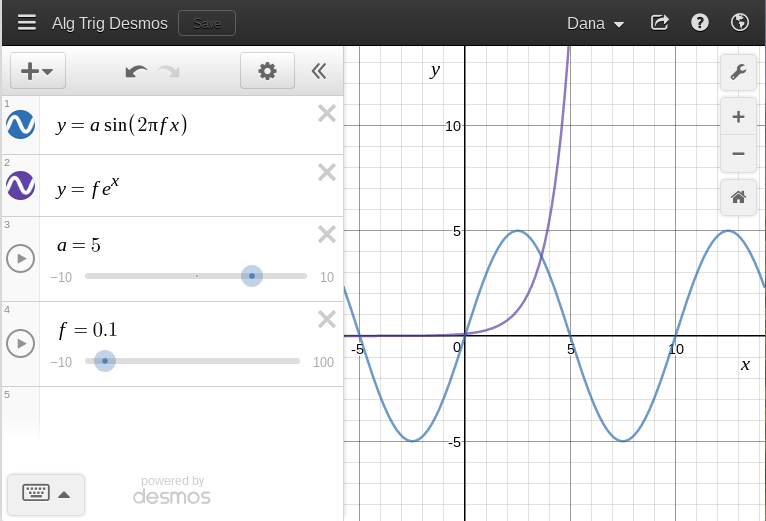This is a page of instructor's scratch work and notes related to the delivery of MS 101 Algebra and Trigonometry summer 2001, 2011, 2012, 2013, 2014, 2015, and 2017, at the College of Micronesia-FSM. These are essentially notes for the instructor, not necessarily for the students, hence the rambling, discombobulated, random nature of these notes. Links may be rotten, step with care.

Spring 2017 these notes were rewritten to utilize Desmos graphing calculator with the intent of replacing the mix of spreadsheets, WolframAlpha, and scientific calculators. The earlier notes remain available. Those earlier notes utilized a software mix consisting of LibreOffice Calc spreadsheets, WolframAlpha, and the use of scientific calculators.

Technical notes: these notes may not validate because the URLs that are produced by WolframAlpha contain bad values for attribute href on element a: Illegal character in query component. The bad values, or "unsafe" characters, are unsafe "because gateways and other transport agents are known to sometimes modify such characters. These characters are "{", "}", "|", "\", "^", "~", "[", "]", and ""." (Berners-Lee, Masinter, McCahill: RFC1738). As these characters are a) fully functional for my non-transport/gateway use, b) an extra step inconvenient step to convert for notes being built in real time on the fly, and c) versions encoded with a URL encoder fail, I simply am not going to fix this issue.

The stucture of the course is to chase a particular model or system each day, and let the math flow from the demands of the model. Models come from a diverse array of fields including genealogy, physical science, health, and web page programming.

## Antecessors and Exponents

An introduction to exponents via ancestry and family treesGenogram from Genopro, a non-free desktop based package.

The diagram above is called a genogram. If you want to make your own genogram, go to FamilySearch.org and start your family tree. You will have to activate an account using an email address. Otherwise you can do this exercise using "pencil and paper"

The course used to use Geni.com, but that site has become increasingly driven to find a way to monetize a relatively small user base. MyHeritage.com is a powerful on line package, but paywalls come quickly, all too quickly for students who can map their family trees out to third cousins and back for multiple generations. FamilySearch does have affiliations to a particular religious community and includes features unique to that faith, but provides the most capability of any free genealogy site. The site also taps into one of the largest genealogy databases on the planet.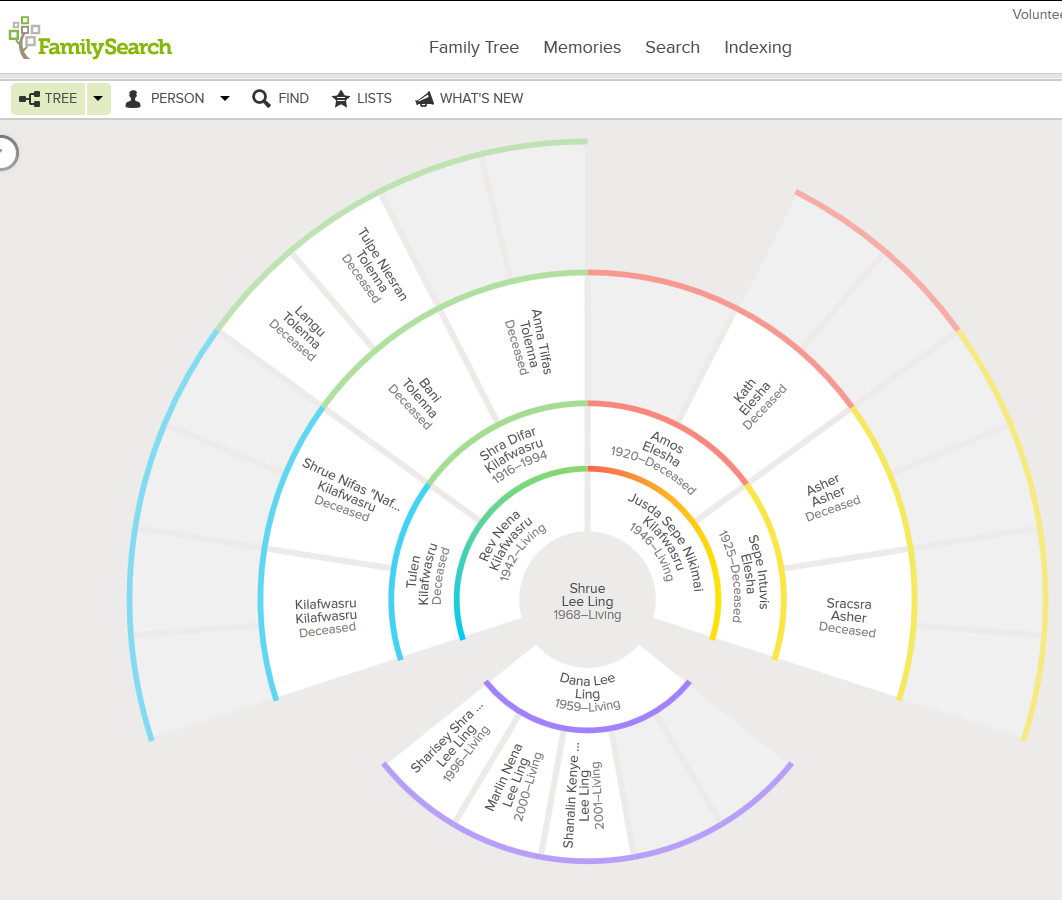1. _____ Generation zero: How many are you?
2. _____ Generation one: How many parents do you have?
3. _____ Generation two: How many grandparents do you have?
4. _____ Generation three: How many great-grandparents do you have?
5. _____ Generation four: How many great-grandparents do you have?
6. _____ Generation five: How many great-great-grandparents do you have?

If the chart extended to generation six, how many great-great-great-grandparents would you have?

Is there a function that can calculate the number of ancestors you have in any given generation?

Obtain historic population numbers

StateYearPopulation
Chuuk192510000
Kosrae1880274
Pohnpei19205000
Yap19208000

### Homework day one

Suppose in every generation each pair of parents has only two children. For example, suppose you have only two children and they each have only two children who also each have only two children, and so forth...

1. _____ Generation zero: How many are you?
2. _____ Generation one: If you have two children, how many children do you have?
3. _____ Generation two: If your children each have two children, how many grandchildren will you have?
4. _____ Generation three: If your grandchildren each have two children, how many great-grandchildren will you have?
5. _____ Generation four: If your great-grandchildren each have two children, how many great-great-grandchildren will you have?
6. _____ Generation five: If your great-great-grandchildren each have two children, how many great-great-great-grandchildren will you have?
7. What function describes the number of children in any generation?
8. What if you have three children who each have three children who each have three children... and so forth. What function would calculate the number of children in generation x?

Graph the function y=2xabove using Desmos. Type y=2^x if using a desktop computer. On a smartphone the keypad includes ab button to enable typing the x in the exponent.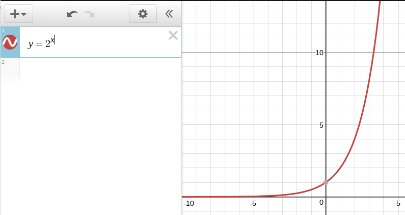The keypad as seen on a smartphone: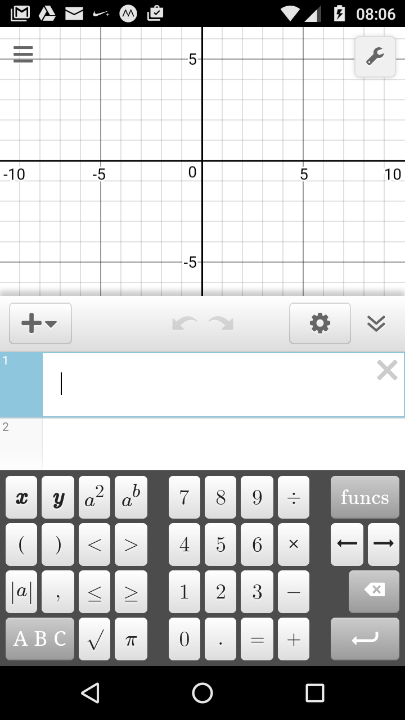Brute force cracking of passwords depends on testing all possible letters, numbers, symbols, for all password lengths, something that is possible using modern graphics card engines.

So in blunting a brute force attack, what matters more, the use of additional symbols or longer passwords/passphrases? Caveat: no memorable string of characters or words will yield a secure password. Only truly random sequences of characters of great length is secure. This exercise is not exploring all means of cracking, only the implications of polynomial growth versus exponential growth in permutations. The greater the number of permutations, the harder the password will be to crack.

What increases the number of permutations more rapidly: adding more characters or increasing the password length? To simplify, let's look at a password with a length of two in which the number of characters increase and then look at a password made of only two characters where the length increases.

Password length 2
Num
1	1		aa
2	4		aa ab ba bb
3	9		aa ab ac ba bb bc ca cb cc
4	16		aa ab ac ad ba ... cd da db dc dd
5	25		aa ab ac ad ae ... ea eb ec ed ee
6	36


As you increase the number of characters, the number of permutations for a given length increases as the square of the number of letters.

Password characters 2
1	2		a b
2	4		aa ab ba bb
3	8		aaa aab aba abb baa bab bba bbb
4	16		aaaa aaab aaba aabb abaa abab abba abbb baaa baab baba babb bbaa bbab bbba bbbb
5	32		aaaaab aaaba aaabb ....  bbbaa bbbab bbbba bbbbb
6	64


As you increase the length of the password, the number of permutations for a given number of characters increases as the power of the number of characters.

The governing function is:
(number of characters in character set)(length of password)

Increasing the characters means that the base changes for baseexponent. The variable is the base, or written algebraically, xconstant. For the example above, x2. This is a polynomial function.

Increasing the length means that the exponent changes for baseexponent. The variable is the exponent, or written algebraically, constantx. For the example above, 2x. This is an exponential function.

Which function increases more quickly and therefore provides the most security? Which matters more - the number of characters or the length?

Desmos can be used to graph the above functions.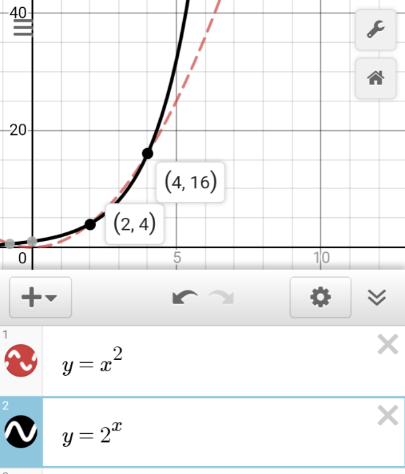Consider the following questions. Does one function always rise faster? What happens between x=2 and x=16? Beyond x=16, which function rises faster? The faster rising function provides more security for a given choice of a trade-off between length and the number of characters. The length of a password is what provides security, not the number of different characters. This is why "pass phrases" are used by some high security sites.

## 5.1 Exponential functions and graphs

Plotting y=2x using Desmos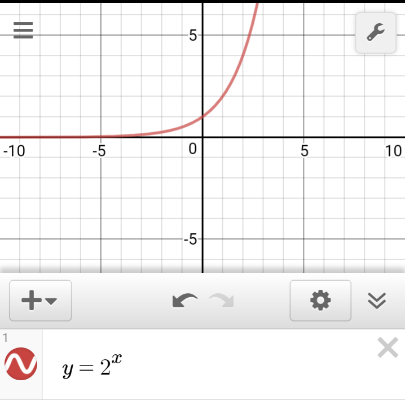To set a specific domain click on the monkey wrench on the graph to access custom settings for the x and y axis, such as setting the domain from −1 to 5 and the range −4 to 32: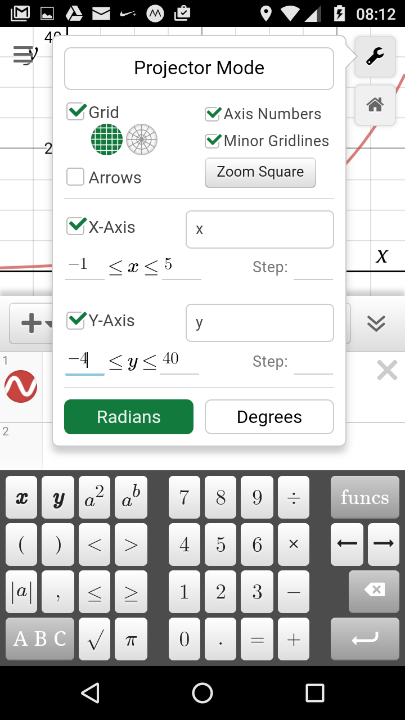Zooming in and out can be done using two fingers on a smartphone. Altering the aspect ratio between the x and y axes can only be done from the axis editor.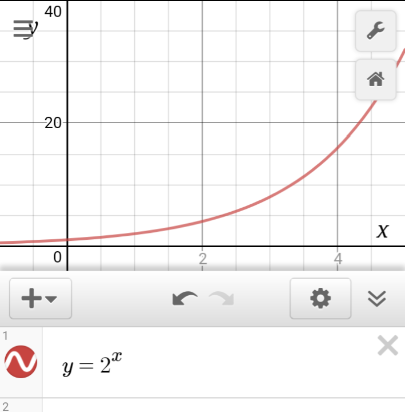To plot multiple functions on one graph add the functions as new lines in Desmos.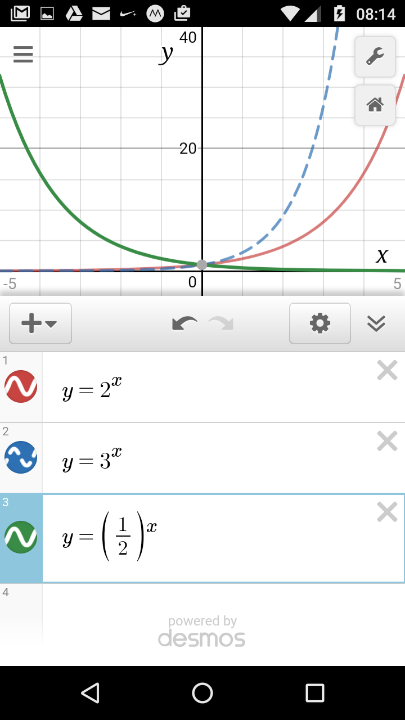### Evaluating a function using Desmos

To evaluate a function such as f(x)=0.9^x at x=1.4 simply type 0.91.4 using the ab button on the keypad to enter the 1.4 in the superscript.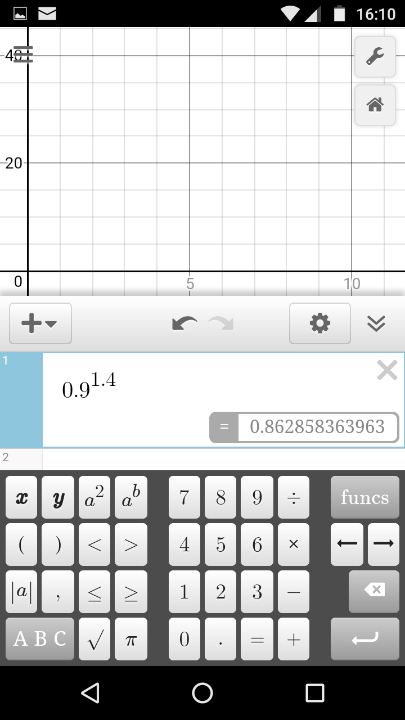Thus Desmos is both a full featured scientific calculator and a graphing engine. Logarithmic and trigonometric functions are available through the Functions button on the keypad.

### Tables of values

Desmos will produce xy scattergraphs from tables. Tables are started from the plus sign.Desmos will accept any single letter variable in the table header. The subscript can be any number 1, 2, 3,... Desmos will start with x1 and y1.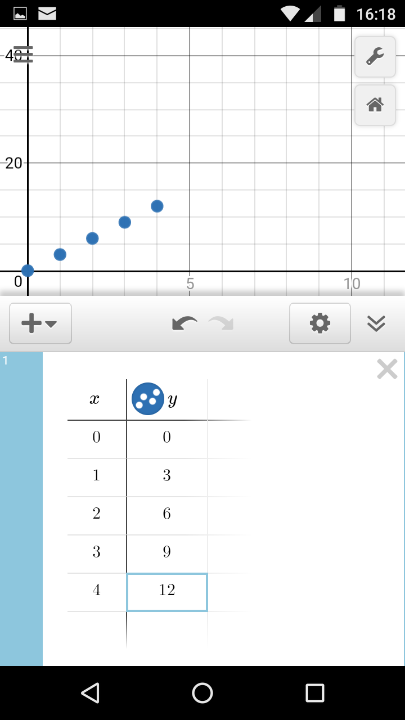Desmos can also generate and graph a table of x-values with the y-values automatically generated by a function.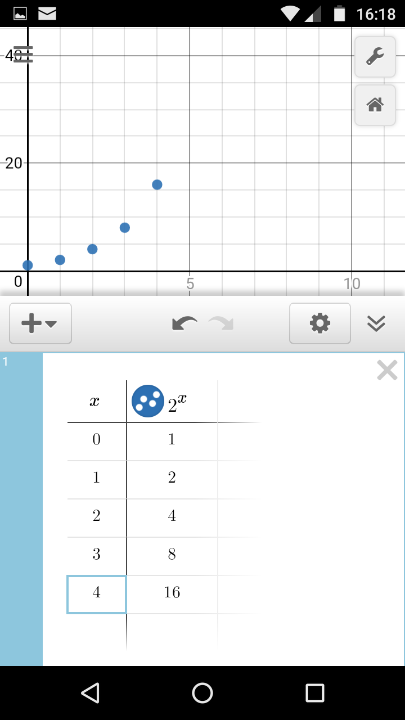### Compound interest

Suppose one wants to change values in a function, evaluating the function at various values, for example the compound interest function. Type in the function with variables in Desmos. Desmos will ask if you want to add sliders. Click on all.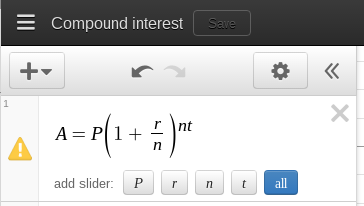The values can then be set to the desired values, the sliders will adjust the endpoints as necessary.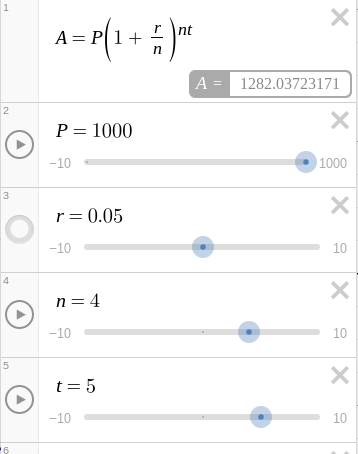Facebook: spread of viral messages, spam. Growth rates at FB average of 130 friends. Growth rate at experimentally determined class average.

SectionEditionPagesProblem set
5.16398-4003, 5, 7-10, 19, 57
7392-3943, 5, 7-10, 11, 67
8388-3909, 11, 13-16, 17, 73

## 5.2 Logarithmic functions and their graphs

Babies! Bring a Chart!

Babies grow logarithmically during the first few months of their life. Finding the function that best models this growth can also be done with Desmos. Note that here I have chosen to change the variables from x1 and y1, the default variables chosen by Desmos, to t1 for the time in months and m1 for the mass in kilograms.

Data table

t1m1
9 3.0
9.5 3.4
11.55.2
13.36.1
14.26.4
15 6.6
17.57.0
21 9.1
25 8.2
26 8.0
27 8.8
37 10.5
57 13.2

Where t1 is the age in months since conception (time)
and where m1 is the mass in kilograms.

Enter the data as a table in Desmos. Then type m1~aln(t1)+b into Desmos. Note the tilde sign! That tells Desmos to find values for the coefficients a and b that best fit the data. You can, if you prefer, enter the implicit multiplication signs, but Desmos takes any single letter that is not a part of a known keyword to be a variable.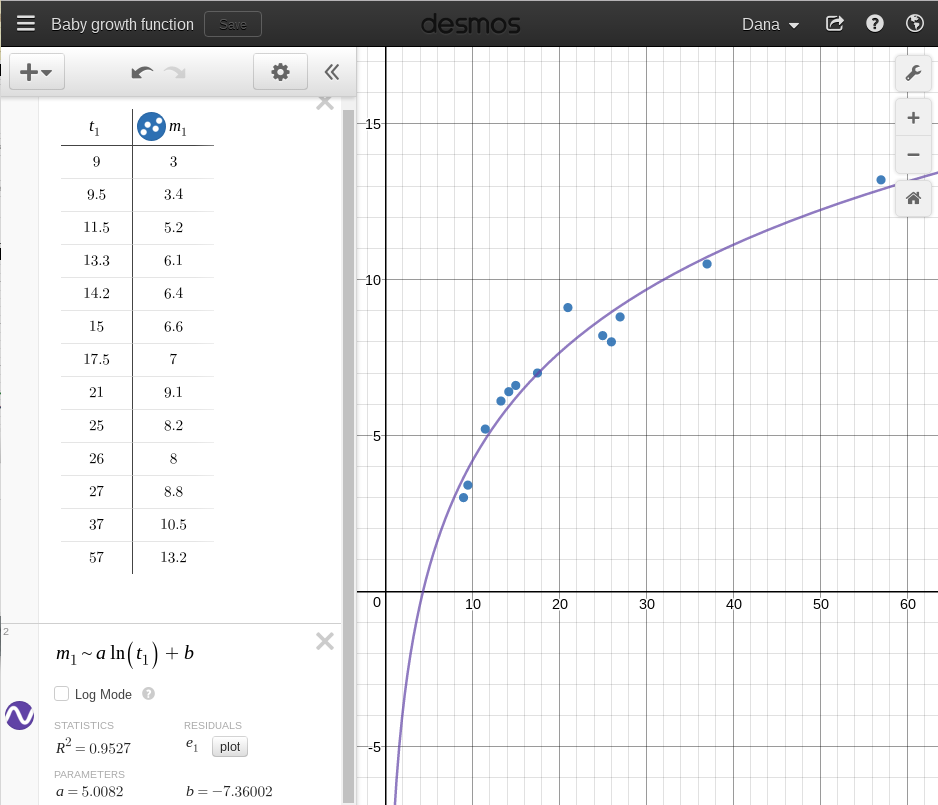(Desmos desktop browser version, smartphone app can also perform regressions)

Thus the function for the growth of the baby is that the mass = 5 ln (time in months) + −7.36. In algebra class we might write this as y = 5 ln(x) − 7.36.

The data is for a baby born in 2000. The dip at 26 and 27 months post-conception was a week long bout of diarrhea that required hospitalization of the baby. Note that "post-conception" is necessary because ln(0) is undefined. Mathematically the age of the baby cannot be zero. The choice of nine months is effectively abitrary.

Desmos includes the natural logarithm function on the keypad. The third tab of the functions button includes ln, log, and loga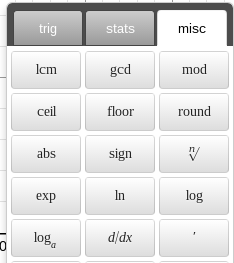These functions can be used in calculations or functions to be graphed.

SectionEditionPagesProblem set
5.274027, 19, 21, 27, 29
839823, 25, 27, 33, 35

## 5.3 Properties of logarithms

The letter e is a special letter in Desmos and is reserved as the base of the natural logarithm. Desmos also includes the exp() function which raises e to the number in the parentheses.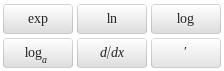As a result, there are two ways to express the base of the natural logarithm.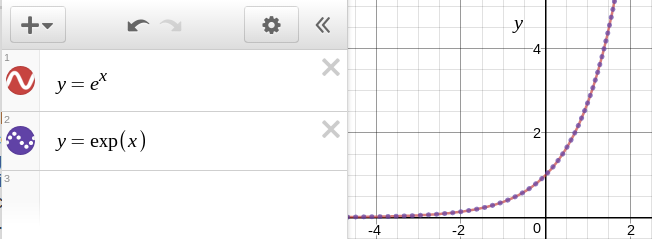### Working in other bases

The Desmos function list includes loga which can be used to calculate logarithms in other bases or to graph logarithms in other bases.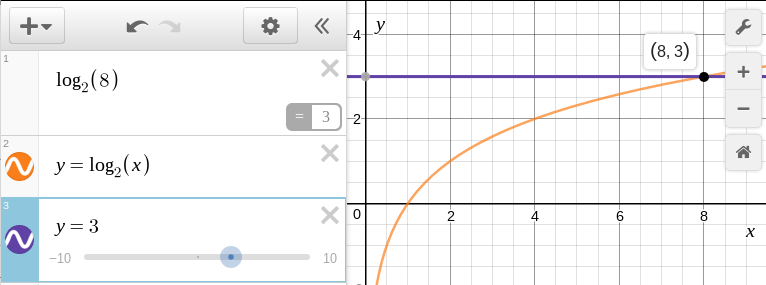Desmos is not an algebraic engine and thus does not expand logarithmic expressions. One can check that two expressions are equivalent by graphing them.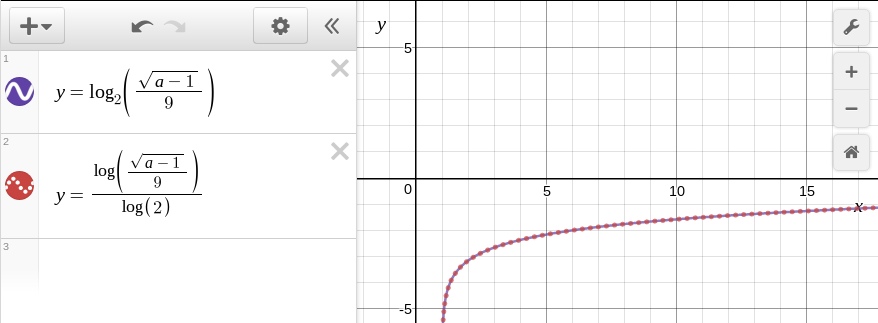Data provided for a galloping speed can be modeled as a y = a + b ln(x) function using the regression capabilities in Desmos.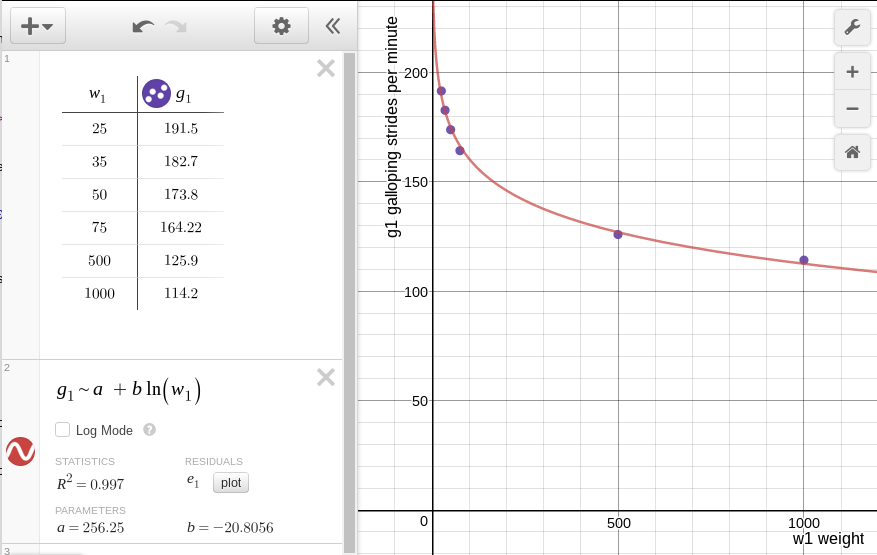Note that Desmos allows one to use letters that are related to the meaning of the variable. In the above example w1 is the weight and g1 is the galloping rate. Do not forget to use the tilde and not an equals sign for regressions!

For reference, the same mathematical result can be obtained in an OpenOffice.org or LibreOffice.org spreadsheet: 5.3 #95 Galloping speed opendocument spreadsheet

Failed example:
Planetary distances from sun
Copy the plain text. Use paste special into LibreOffice.org and merge the space delimiters. Getting fancy: include the | delimiter. Select and sort on AU distance. Dead end. Leads to exponential. Can use log to linearize, but text fudges AU distances to improve fit. Plus the text book does not return to the logarithm as a mathematical explanation.

WolframAlpha will expand logarithms using the expand command, but will not "condense" (textbook's word) logarithms. Attempts to use simplify and combine also failed to produce the "condensed" result.

SectionEditionPagesProblem set
5.37409 9, 11, 13, 15, 23, 25, 29, 85
840515, 17, 19, 21, 29, 31, 35, 95

## 5.4 Exponential and Logarithmic equations

Desmos can provide graphical solutions to problems that do not at first appear to be graphical.

${e}^{{\mathrm{-x}}^{2}}={e}^{\left(-3x-4\right)}$

Desmos does not appear to algebraically solve the equation, yet the answer is displayed as vertical lines at the solutions.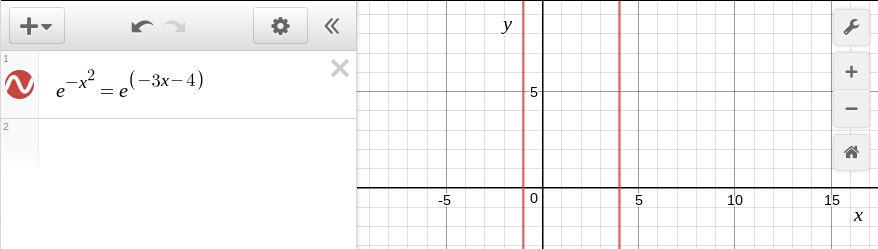A more nuanced view of the solutions is available by displaying the separate functions. Entered this way, one can click on the intersections to display the coordinates of the intersections. The solutions to the original problem are the x values.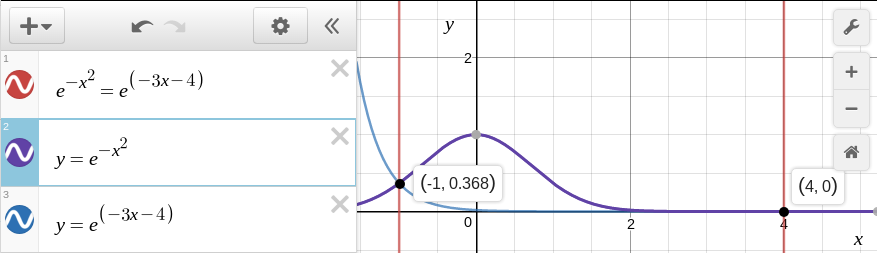The solution on the right side can be examined more closely by adjusting the domain and range for the graph.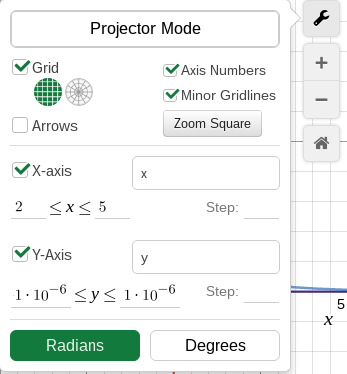The result is a view of the exponential behavior of these functions along the x-axis at any scale.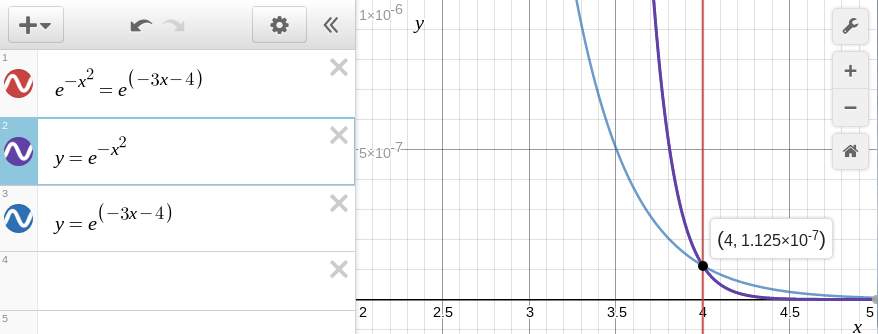Solving log(5x)+log(x-1)=2 can proceed using either of the paths shown above: enter as shown or enter the corresponding functions for the left and right side.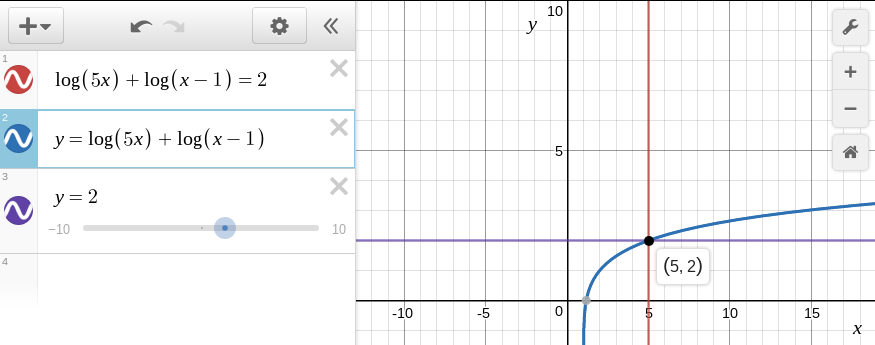Clicking on the intersection displays the solution. Note that a graphical analysis does not produce the spurious solution that working the problem by hand generates.

8th edition p417 #137. The automobiles problem, part a:

Desmos makes setting up the table using the specified easy. First enter the x values.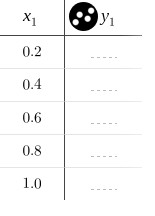Then enter the function, remembering to use x1 for the variable. The y-values appear automatically.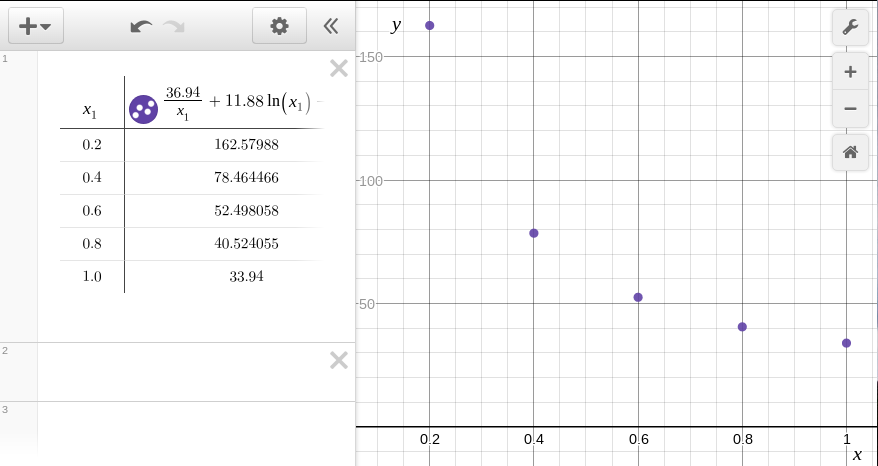Desmos can display those as circles, a smooth line through the points, or a smooth line with circles.

## 5.5 Exponential and Logarithmic models

Chose to focus on Usain Bolt's 2008 100m world record. At 8:00 in the morning there is still enough bandwidth to show an on line video - sort of. So I found and showed a video of Usain Bolts run, not a very good one, but just to give the class an idea of what happened.

Then I had the students enter Usain distance versus speed data into a spreadsheet and then try the four regression models in LibreOffice.org to find a fit.

Distance (m)Speed (m/s)
00.0
105.4
209.8
3011.0
4011.5
5011.8
6012.2
7012.2
8012.2
9012.1
10011.1

None of the four models fits well, although the students favored the logarithmic fit. The logarithmic model may be a best fit, but the function cannot handle x = 0, starts too fast, and, possibly most problematic, the speed continues to rise without limit. Sprinters hit a maximum speed and then attempt to hold that speed into the finish line. The logarithmic model simply behaves incorrectly.

Desmos is the game changer here. Desmos will regress to any arbitrary function. In the table and graph shown, the distance d1 versus the velocity v1 if regressed against a logistic function. The result is very close to the coeficients that were estimated years earlier. There is no need to use the EXP function, Desmos uses straight mathematical notation.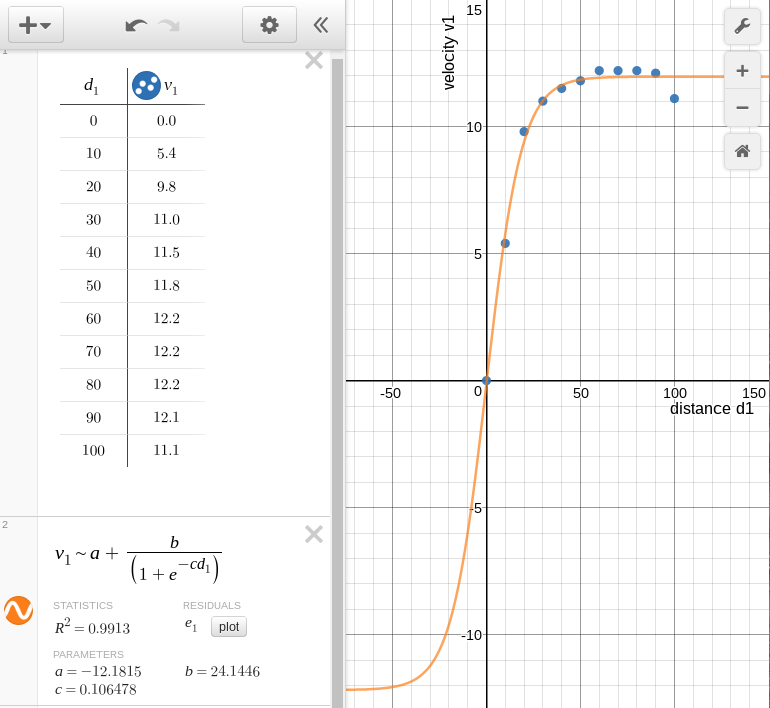Homework can include Usain's speed at 15 meters and the distance at which Usain reaches my running speed, 2.5 m/s.

In physical science a measurement is made of height versus velocity for a freely rolling RipStik rolling from a height h1. The velocity is v1.

where h1 is the height in centimeters and v1 is the velocity at the bottom of the slope in centimeters per second.

The theory is that ${v}_{1}=44\sqrt{{h}_{1}}$. Unlike marbles, RipStiks do not "spin" hence the angular momentum term, although technically true for the RipStik wheels, is removed. Spreadsheets cannot regress to an arbitrarily specified function. The only option is to use a power function in a spreadsheet.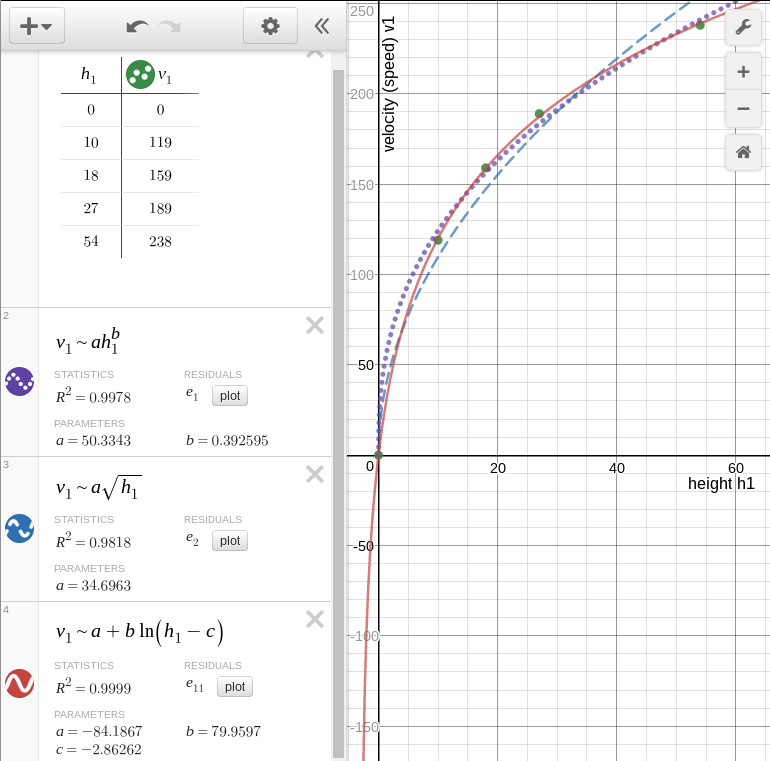The power function produces the dotted line seen on the graph. One is left arguing that the value of b (0.39) is reasonable close to 0.5 and that 0.5 is a square root relationship. Desmos, however, allows one to regress to any arbitrary function, including a square root relationship with a single coefficient and no intercept. The result is far easier to explain. The 35 is less than 44 due to friction, but the shape supports the theory. Alternatively, one can regress to a logarithm and achieve an even better fit. Note that to avoid an undefined value at zero height a constant c is subtracted to permit the origin to translate. This permits the data to be used in exponential and logarithmic models. Note too the ability of Desmos to simultaneously display three different regressions.

## 7.1 Angles and arc lengths

Concept is to hope for sun and run three radii of yarn out. Get angle in degrees. Use surveyor's wheel to measure actual arc length. Calculate radian measure.

Summer 2012 measured a 180° arc on the lawn with a radius of 1145 cm. Surveyor's wheel arc was 3627 cm (119 feet). Surveyor's wheel kept tending to go straight along a chord of the circle, slacking the radial line. Angle is pi, 3.16 by the above numbers.

Summer 2013 rain forestalled going outdoors. I borrowed a wheel used to demonstrate the gyroscopic effect. The wheel had a radius of 25.5 centimeters. I cut poster paper in half the long way, taped the narrower ends together, and placed the paper on two folding tables placed end to end. I put four evently spaced post-it notes on the wheel rim. Using a cloth tape measure, I determined the wheel to have a circumference of 160.2 cm, which I rounded to 160 cm. I rolled the wheel and marked the post-it note locations. The post-it note marks were not at exactly 40 cm, so I used the 160/4 = 40 cm to calcuate the angles. 40/25.5 = 1.57. 80/25.5 = 3.14. 120/25.5 = 4.71. 160/25.5 = 6.27.

I noted that these number had no units. When dividing 160 cm ÷ 25.5 cm, the centimeters cancels. These angles have no measure, no units. Only later did I introduce the term radians.

Then I introduced the degree based system. There are discussions of why the degree system uses 360 degrees in a circle in the Wikipedia and in the Math Forum.

I should have, but did not get time to, put up a unit circle with both radians and degrees.

Summer 2015 a 44 foot length of heavy clothes line and a surveyors wheel was used to measures distances around a circle. $\frac{\pi }{2}$ was at 68 feet of arclength. π at 145 feet of arclength. $\frac{3\pi }{2}$ at 216 feet. 2π at 295 feet. π a tad high at 3.35

Vertex, angles, acute 30°, 45°, 60°, right 90°, complementary, obtuse, straight 180°, supplementary. θ.
s= rθ.
Rotational velocity ω

Desmos can operation in radians or degrees, this is set from the coordinates dialog box available via the monkey wrench icon.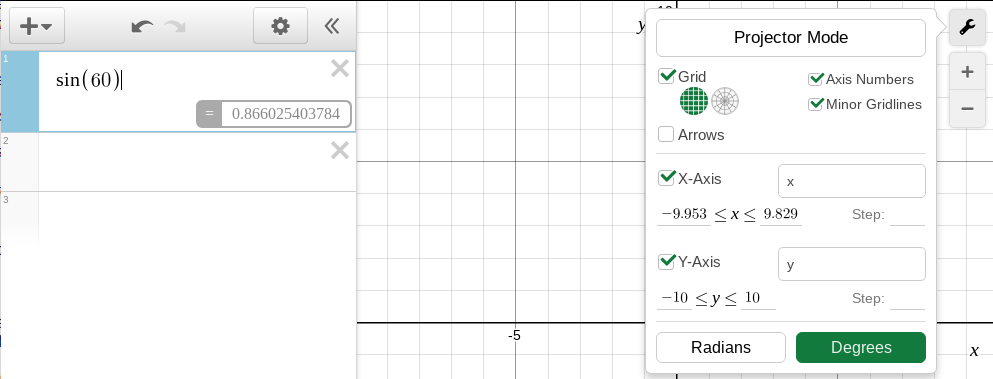Desmos also has a scientific calculator that can be set in degrees or radians. This is a scientific calculator on the same steroids as Desmos' graphing calculator. The scientific calculator handles the same functions as the graphing calculator, and the notation used conforms to mathematical usage.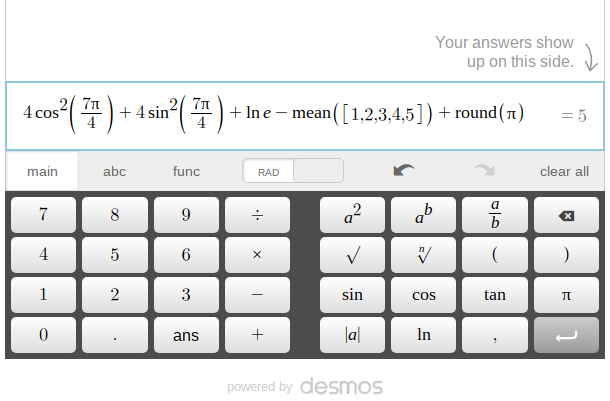A geometric constructions scratch pad is under development by Desmos.Desmos geometry does not have angle input functionality, obtaining a right triangle requires inscribing the triangle in a circle. The scratch pad features "snap to" existing lines that aids in constructions.

Note: The above homework is easier and faster with a calculator and the relationships pi radians = 180° and s= rθ

## 7.2 SVG

Part way through this unit during summer 2017 I realized that there would exist the option of doing all of this in Desmos. The same work with shapes could and should be done entirely in Desmos. BUT... getting the sides of the pentagon is a slope-intercept to point slope frightmare. Worthy frightmare.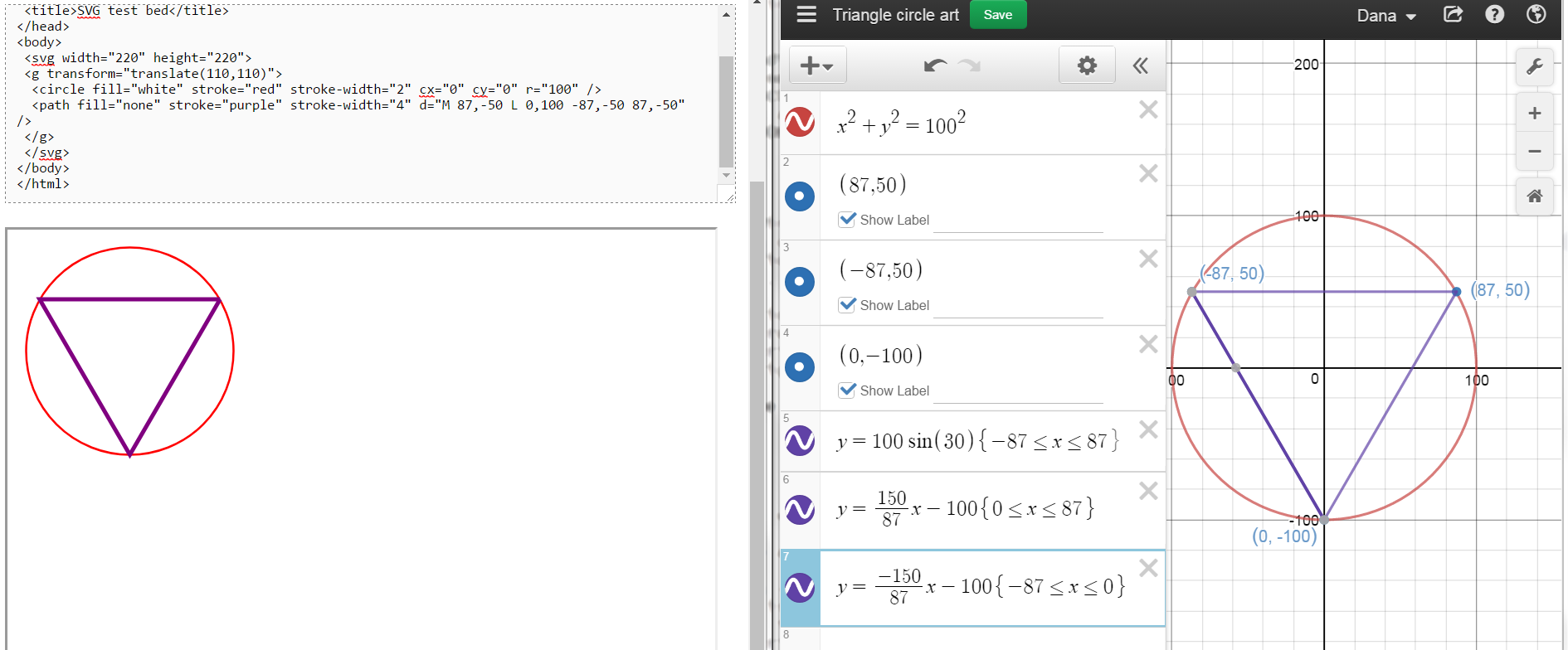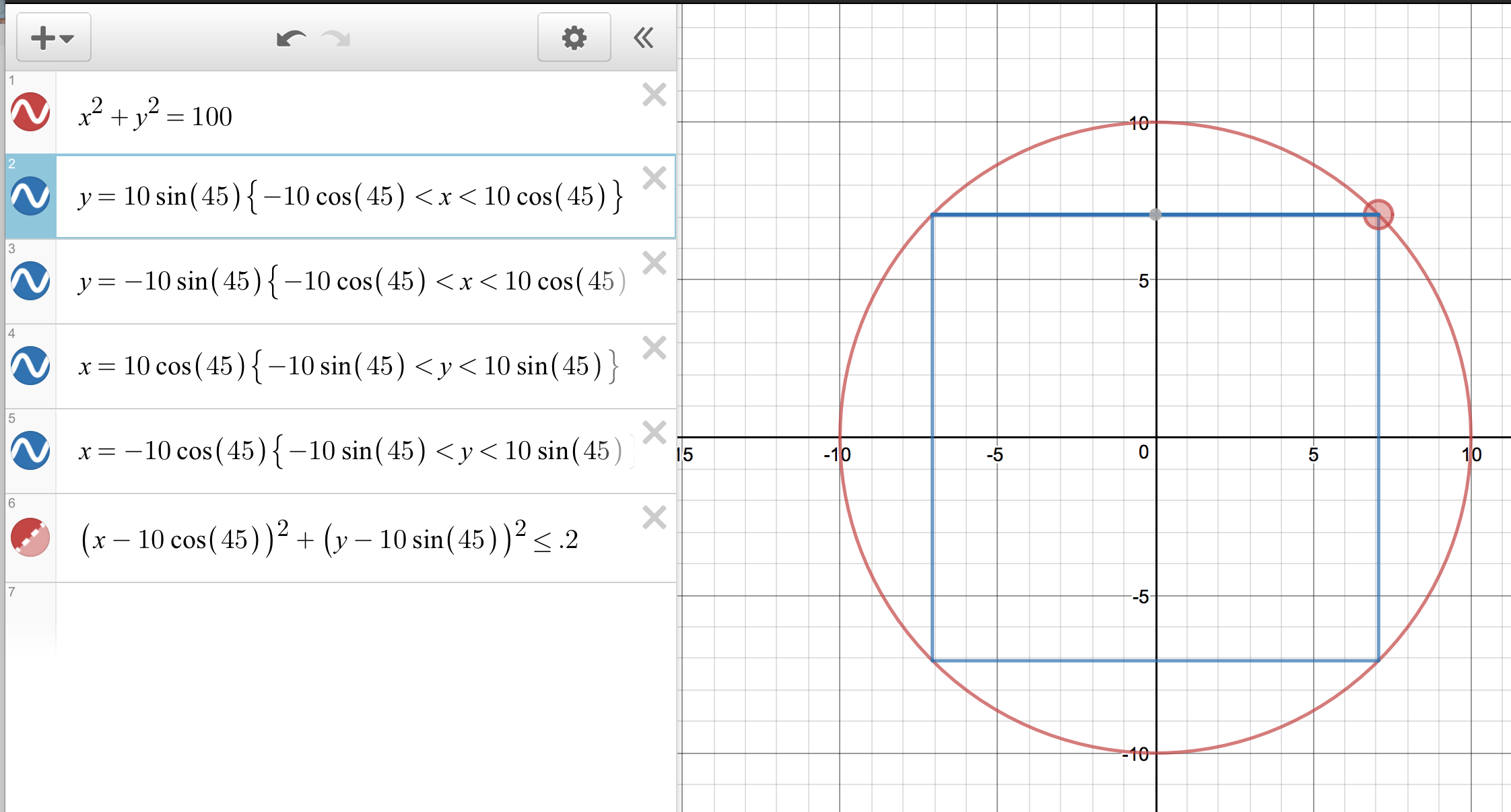Over the next couples of days the models focus on web page creation using Scalable Vector Graphic (SVG) extensions to HTML5. This is the cutting and bleeding edge of web page design. You will be using trigonometry to lay out some basic shapes on a 200 by 200 grid with these technologies. These skills, based in mathematics, are of most use to the CIS majors. The activities also provide a vehicle for exploring the trigonometric functions.

SVG graphics load with the web page because they are simply HTML text based code. This results in fast load times. The graphics are also easy to reuse, modify, and redeploy because they are simply text. SVG graphics also rescale without "jaggies" developing. The following is an example of an SVG graphic.

### SVG coordinate system

Note that a transform="translate(100,100)" statement is used in the SVG to move the origin from the upper left corner to the center.

The SVG grid is like an xy graph EXCEPT that the y-axis is UPSIDE-DOWN. To draw shapes on the grid you will use a text editor. This will be demonstrated in class. Then the pages will be opened and viewed using FireFox. As of 2013 Google Chrome can also render SVG, but not MathMl. These notes are composed in HTML5 using both SVG and MathMl.

HTML5 + SVG code is composed using a text editor.

### Windows

In Microsoft Windows use Notepad.exe as a text editor.Launch this from the "Run" dialog box in the start menu.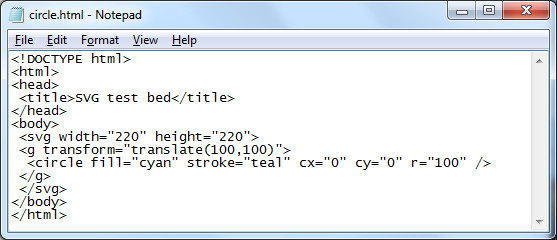### Ubuntu

The text editor in Ubuntu has to be opened from the activities button in the top left corner of the screen to open the launchers menu. Click on the More apps button and start typing "text editor".Click on the text editor. To help check for errors, set the highlighting mode to HTML.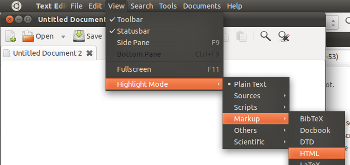### Lubuntu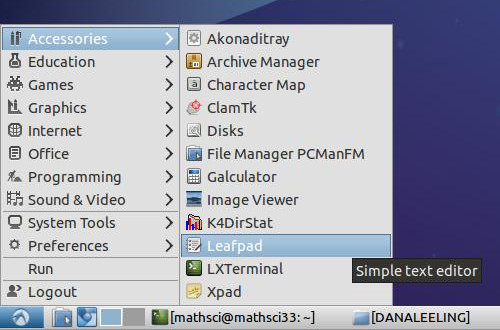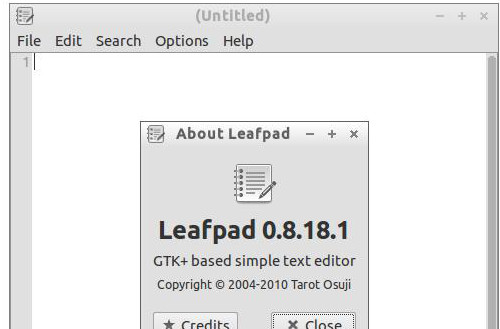For more capabilities, download and use SciTE. SciTE is in the Lubuntu repositories.

### ChromeOS

In ChromeOS, use Caret app from the Chrome Web Store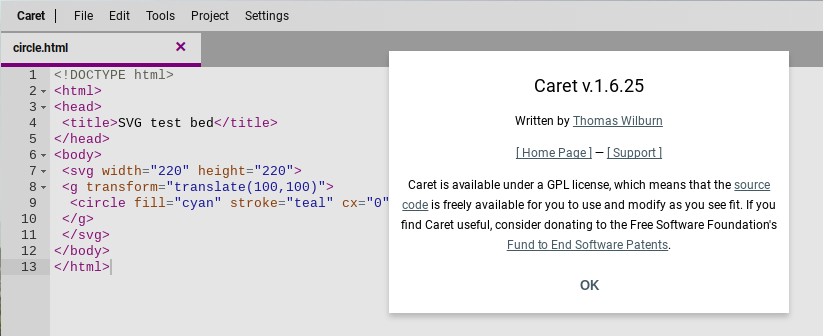### Coding

The following HTML code will draw a circle with a radius of 100 pixels on a web page.

<!DOCTYPE html>
<html>
<title>SVG test bed</title>
<body>
<svg width="220" height="220">
<g transform="translate(100,100)">
<circle fill="cyan" stroke="teal" cx="0" cy="0" r="100" />
</g>
</svg>
</body>
</html>


HTML is very very fussy - the slightest typing error will cause the web page to fail. The center of the circle is at (cx,cy). The numbers are measurements in pixels. Pixels are "picture elements". Picture elements are the smallest controllable element of a computer monitor.

The next task is where trigonometry comes into play: we are going to draw a square in the circle. To draw the square exactly inside the circle ("inscribed") we need to determine where the corners of the circle are located. The circle has a radius r of 100.

To draw a square in a circle we will need to locate the corners of the square. To do this we will need to work out the length of the adjacent and opposite sides of a 45° triangle. This will help us locate the corners of the 45-45-90 right triangle that is the building block of the square.

The SVG code to draw this square will have the form of...

<path fill="none" stroke="silver" d="M x1,y1 L x2,y1 x2,y2 x1,y2 x1,y1" />

where the x1,y1 elements will be replaced by numbers, by coordinate pairs.

Calculating the coordinates requires determining the length of the bases of the 45-45-90 triangle. This calculation requires using sine and cosine. The sine function, abbreviated sin, can be used to calculate the length of the side opposite the angle theta θ.

Desmos launches in radians mode by default. Switch to degrees mode.

$\mathrm{sine}\left(\theta \right)=\mathrm{sin}\left(\theta \right)=\frac{\mathrm{opposite}}{\mathrm{hypotenuse}}=\frac{y}{r}$

Cross multiply and one gets y = r sin(θ).
r = 100 and θ = 45°
Thus y = 100*sin(45°)

The cosine function, abbreviated cos, can be used to calculate the length of the side adjacent the angle theta θ.

$\mathrm{cosine}\left(\theta \right)=\mathrm{cos}\left(\theta \right)=\frac{\mathrm{adjacent}}{\mathrm{hypotenuse}}=\frac{x}{r}$

Although we are not going to use tangent function at the moment, there is a third trigonometric function, the tangent function.

$\mathrm{tangent}\left(\theta \right)=\mathrm{tan}\left(\theta \right)=\frac{\mathrm{opposite}}{\mathrm{adjacent}}=\frac{y}{x}$

For direct variation, a direct relationship where y=mx and the y-intercept is zero, the tangent is the slope of the line. The tangent function provides a connection between an angle θ and the slope of a line at that angle.

There are also reciprocal functions, these will not be that directly useful to our work.

The three relationships are sometimes memorized using "SOHCAHTOA": Sine is Opposite over Hypotenuse, Cosine is Adjacent over Hypotenuse, and Tangent is Opposite over Adjacent.

If the hypotenuse is taken to be the radius r, and if the adjacent is taken to be x, the opposite taken to be y, then sine θ = y/r, cosine θ = x/r, and tangent θ = y/x.

Cross multiply cosine θ = x/r by r and one gets x = r cos(θ). r = 100 and θ = 45° Thus x = 100*cos(45°) To calculate this use WolframAlpha: 100*cos(45 degrees)

For both of the above results, we will have to round off the decimal portion of the number. SVG does not use fractional pixel values. Both calculations have the same answer: 71 pixels.

The center of the circle is (0,0). This is our "starting" place. Moving to the right 71 pixels adds 71 to x-value in the (x, y) coordinate. This puts us at the right-angle corner of the triangle.

Moving up 71 pixels is moving in the negative y direction. This means we have to subtract 71 from the y value at the right-angle corner.

Thus the coordinate for the upper right hand corner is (71,-71). This will be the first coordinate in the path command that will draw our square

<path fill="none" stroke="black" d="M 71,-71 L , , , , " />

The "M" in the path attribute means "Move to..." This tells the computer where to start our SVG diagram. The "L" means "Line" as in "draw a Line to". The next step is to tell the computer where the line should go.

Let us start by going down to the lower right corner of the square. Using the same basic argument as above, the lower right corner of the square must be 100*sin(45 degrees) below the center - that is, 71 pixels down from 0. Or 142 pixels down from the upper right corner. Each side of the square is two times 71 pixels long.

<path fill="none" stroke="black" d="M 71,-71 L 71,71 , , , " />

Using the above approach, we can work our way around the square, corner to corner, returning eventually to the upper right corner at (71,-71).

<path fill="none" stroke="black" d="M 71,-71 L 71,71 -71,71 -71,-71 71,-71" />

Square, re-centered on (0,0)

<!DOCTYPE html>
<html>
<title>SVG test bed</title>
<body>
<svg width="220" height="220">
<g transform="translate(100,100)">
<circle fill="lavender" stroke="indigo" cx="0" cy="0" r="100" />
<path fill="thistle" stroke="black" d="M 71,-71 L 71,71 -71,71 -71,-71 71,-71" />
</g>
</svg>
</body>
</html>


The colors used in the above HTML/SVG code come from the X11 color palette.

### Equilateral triangle

Summer 2013 I began with a hexagon inscribed in a circle and then drew in the equilateral triangle with one vertex due west. I used a circle center of (0,0) and a radius of 100 after showing that a radius of one pixel was too small to be usefully seen. I used a (0,0) center and added a transform="translate(100,100) to move the diagram to the correct center. This increased the "symmetry" of the path points. The complication is that introduces negative coordinate values. Negative numbers can lead to sign errors, so I do not know if the simplification of the coordinates via symmetry will be offet by the complication of negative coordinate values. The new (0,0) center led to considerable symmetry in coordinate values. The notes were post hoc updated in 2013 to begin from a transformed (translated) coordinate system. In 2014 and 2015 the transformed coordinate system was used. There are both gains in symmetry and a natural logic to the circle being centered at (0, 0). The original untranslated coordinate system has not been used since 2012.

Next we will tackle an equilaterial triangle. The key to solving this will be using the 30-60-90 triangle in the diagram below.

Summer 2012 layout on the left. Summer 2013 layout as of the end of Monday 24 June on the right. Summer 2013 the center was set at (0,0). On Tuesday I will introduce the transform attribute on a graphics element g to move the triangle: <g transform="translate(100,100)"> and </g> The notes below are not yet edited to reflect this shift. Homework is to find the coordinates of the red circles based on the center being at (0,0)

Homework: Using adjacent = r cos θ and opposite = r sin θ, try to work out the coordinates of the circle at the vertices of the equilateral triangle. Note that r = 100, the center of the triangle is located at (0, 0), and the angle θ is 60°. The grid we are using is on the right.

#### Tuesday

The angle θ is 60°. Your text book notes that a 30-60-90 triangle is another "special" triangle. They are special because they build equilaterial triangles and hexagons, among other reasons.

The hypotenuse r is 100 pixels long. The angle θ is 60°.

The adjacent side uses the cosine function:

$\mathrm{cosine}\left(\theta \right)=\mathrm{cos}\left(\theta \right)=\frac{\mathrm{adjacent}}{\mathrm{hypotenuse}}$

adjacent = r cos(θ). r = 100 and θ = 60° Thus adjacent = 100*cos(60°) To calculate this use WolframAlpha: 100*cos(60 degrees)

The opposite side still uses the sine function:

$\mathrm{sine}\left(\theta \right)=\mathrm{sin}\left(\theta \right)=\frac{\mathrm{opposite}}{\mathrm{hypotenuse}}$

opposite = r sin(θ). r = 100 and θ = 60° Thus opposite = 100*sin(60°) To calculate this use a calculator or WolframAlpha: 100*sin(60 degrees)

The adjacent side is 50 pixels. This can be added to the appropriate value at the origin. Think of moving 50 along the adjacent side.
Rounded off to the nearest whole number, the opposite side is 87 pixels. This tells us to go 87 from where we ended up after moving 50 along the adjacent side.

Whether the 50 is added to the x or y value of the center depends on the orientation of the triangle. The summer 2012 "point north" triangle has the 50 added to the y-value. The summer 2013 "point west" triangle adds the 50 to the x-value of the center. Work out the appropriate values and additions/subtractions from the diagram.

As you "work" your way around a diagram always remember: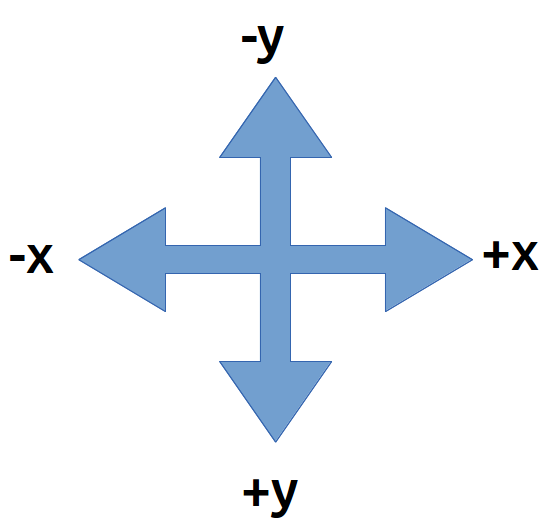Summer 2012 path element code:

<path fill="none" stroke="black" d="M 100,0 L 187,150 13,150 100,00" />

Homework summer 2012: Draw an equilateral triangle with one vertex at the bottom of the square.
Homework summer 2013: Draw an equilateral triangle with one vertex pointing east.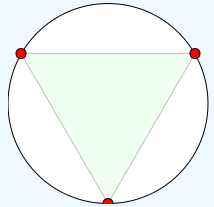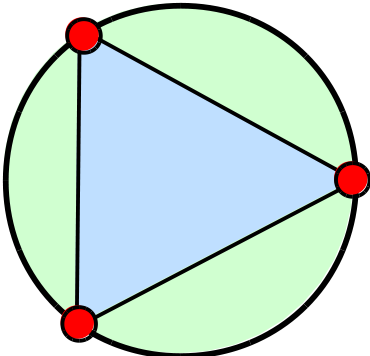The relationship between x, y and the angle theta θ is given by a third trigonometric function, the tangent function. The tangent is also the slope of a line if the adjacent side is horizontal.

$\mathrm{tangent}\left(\theta \right)=\mathrm{tan}\left(\theta \right)=\frac{\mathrm{opposite}}{\mathrm{adjacent}}=\frac{y}{x}=\frac{\mathrm{rise}}{\mathrm{run}}=\mathrm{slope}$

SectionEditionPagesProblem set
6.374791ab, 65, 67, 69
84755ab, 71, 73, 75, 107, 109

The following will display in FireFox 6.0a1 (Mozilla/5.0 (Windows NT 6.1; Win64; x64; rv:6.0a1) Gecko/20110523 Firefox/6.0a1). The html will not pass validation - no doctype is the primary error.

<html>
<title>SVG test bed</title>
<body>
<svg fill="turquoise" stroke="teal" >
<circle cx="20" cy="20" r="10" />
</svg>
</body>
</html>


But oddly enough the following actually validates!

<!DOCTYPE html>
<html>
<title>SVG test bed</title>
<body>
<svg fill="turquoise" stroke="teal" >
<circle cx="20" cy="20" r="10" />
</svg>
</body>
</html>
`

The above, however, is limited in FireFox to some sort of default vertical height of 150. SVG elements must explicitly close or subsequent elements fail.

The new text has material in different chapters and sections. Even as the notes get updated, the links from the syllabus become broken.

## 8.1 Graphs of trigonometric functions

I used my RipStik to introduce section 8.8. Getting a good sine wave requires traveling in a perfectly straight line at a constant swizzle rate and a constant linear speed. I used too short a run in and was not set up and moving stably as I crossed the paper. The next day in physical science class I happened to be running the same exercise for section 091. I found that if I used over half the porch to set up my swizzle and my speed, I could run a very straight line with a clean swizzle. I used all of the length of the A201 and A202 classrooms, crossing the paper midroom A203.

Introducing Sine Waves in Trigonometry with a RipStik Larson notes: Using this approach I also introduced the wavelength - something the text omits in favor of focusing on the period. I also introduced frequency, something the text leaves for a homework problem (77 in the seventh, 91 in the eighth). I brought in a tuning fork and showed the class that Larson has the problem the wrong-end around: tuning forks always have their frequency stamped on the them, not their period. One can then work out the period from the frequency.

OpenStax notes: This text also forgoes mention of wavelength and frequency.

When entering trigonometric formulas into Desmos, typing "pi" produces π.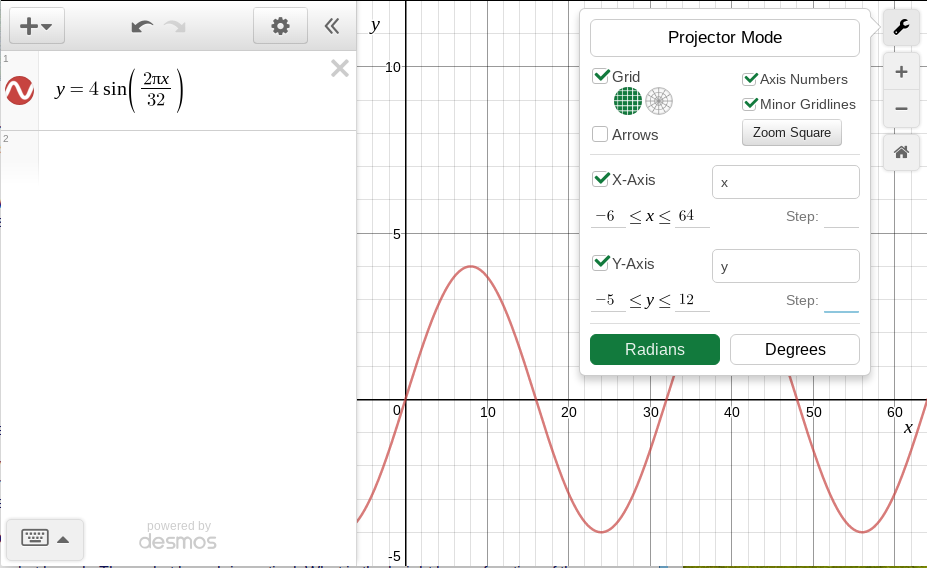The WolframAlpha approach: plot 4 sin(2*pi*x/32) from 0 to 64

I also worked the wavelength from the relation wave velocity = frequency * wavelength and the speed of sound (http://www.wolframalpha.com/input/?i=sound+speed+32+Celsius+74%25+relative+humidity yields about 350 m/s. Data is from my physical science class.)

SectionEditionPagesProblem set
6.474901, 2, 3, 23, 24, 57, 77
84865, 7, 9, 27, 28, 67, 91

## [Larson 6.5]

OpenStax is arranged differently. I opted to use the day after OpenStax Alg and Trig 8.1 to demonstrate sound waves, constructive interference, Android apps such as Keuwlsoft's offereings, and on line tone generators and oscilloscopes for use by teachers in teaching about sound waves.

## 8.3

The sine function does not pass the horizontal line test, thus constructing a inverse function is problematic. However, by restricting the domain to -90° ≤ x ≤ 90°, then an inverse function can be defined. The inverse function of the sine calculates the angle θ from the value of the opposite divided by the hypotenuse.

Desmos produces angles in radians when given an inverse trigonometric function. When set to degree mode, Desmos will return degree values. Students should check which mode they are in as they work problems.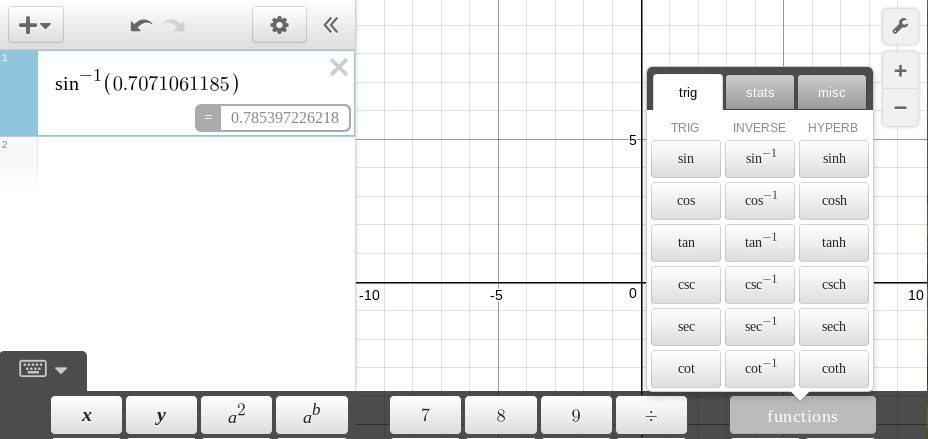Given an angle in a triangle with a hypotenuse of 5 and an opposite side of 3, what is the angle θ?

Pythagorean triples the fun way: Have two different students pick two different numbers a and b. Then calculate (a+bi)(a+bi). The resulting b+ci complex number are the legs of a pythagorean triple triangle with leg lengths b and c. Learned that from a YouTube video.

Use the triples in the search for the angles for those triangles

I then noted that the next step was one that was focused on the CIS majors: using a mixed address formula in a spreadsheet to generate a map of Pythagorean triples. For a list sorted by angles closest to 45° see pythagorean triples list.

Homework is now entirely inside Schoology. Remnant diagrams from the past. The angles θ and φ for the following triangle:

and find the length of the hypotenuse and the angles θ and φ for:

## 9.5

9.5 Solving Trigonometric Equations

Lead off with re-explaining how this works. Start with 3x=9, move to x^2+8x=-15 and then use that to show domain restriction option. Cover range for sine, cosine, tangent, in both degrees and radians. Then move on to trig equations and their solutions. The beauty of Desmos is that one can see the behavior of the function in a way that abstract solving does not permit.

For the expression:

$\frac{1}{12}cos\left(8t\right)-3sin\left(8t\right)=0$

where 0 ≤ t ≤, use a technique of setting each side equal to y. Note that the curly brackets specify a domain. The graph bounds were also adjusted to the domain and range.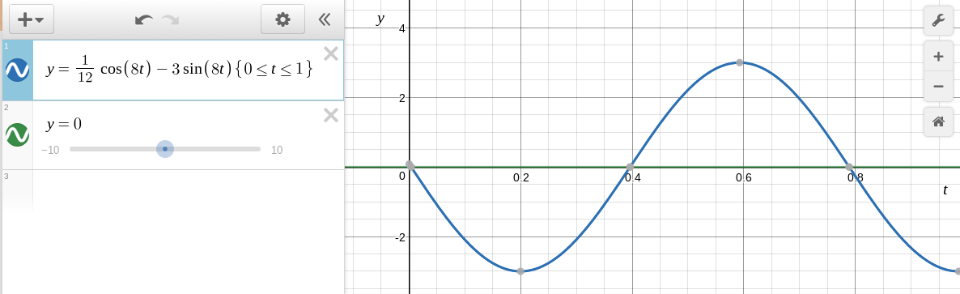## OpenStax Algebra and Trigonometry 10.3 Polar Coordinates

10.3 Polar Coordinates

Where in xy coordinates we plot pairs at (x,y) on a rectangular grid, in polar coordinates we plot pairs (r, θ) on a circular grid. Note that θ is an angle in radians.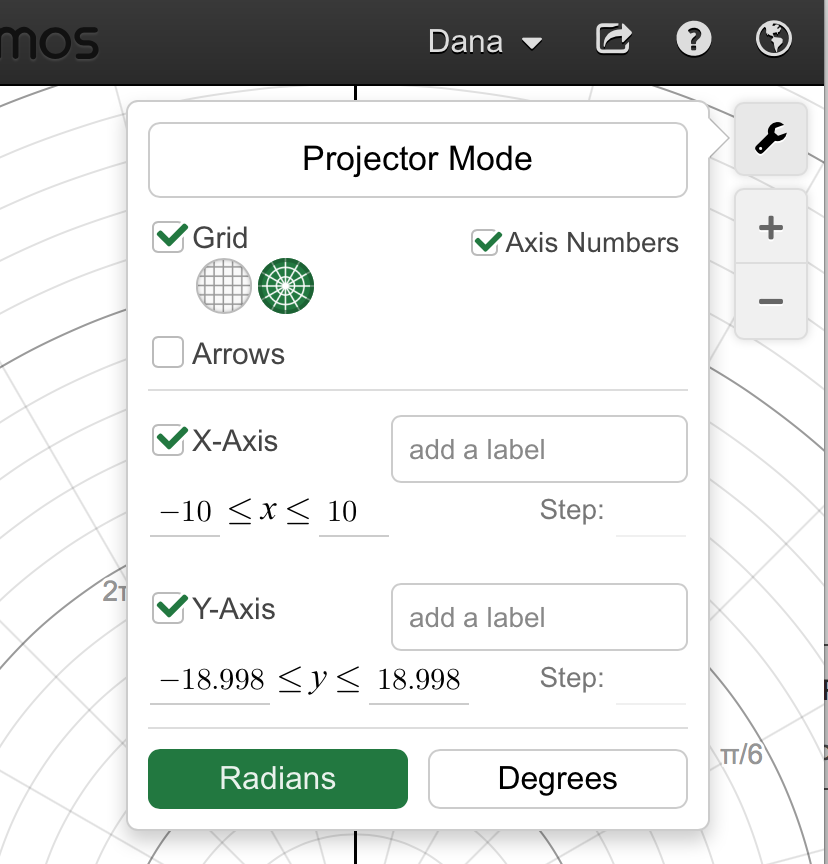Conversions:

r and θ to x and y

x = r cos θ
y = r sin θ

x and y to r and θ

r = SQRT(x² + y²)
θ = arctan(y/x) = tan-1(y/x)

## 10.4 Graphing on the polar grid

Polar coordinates r and θ and art. Flowers on the polar plane.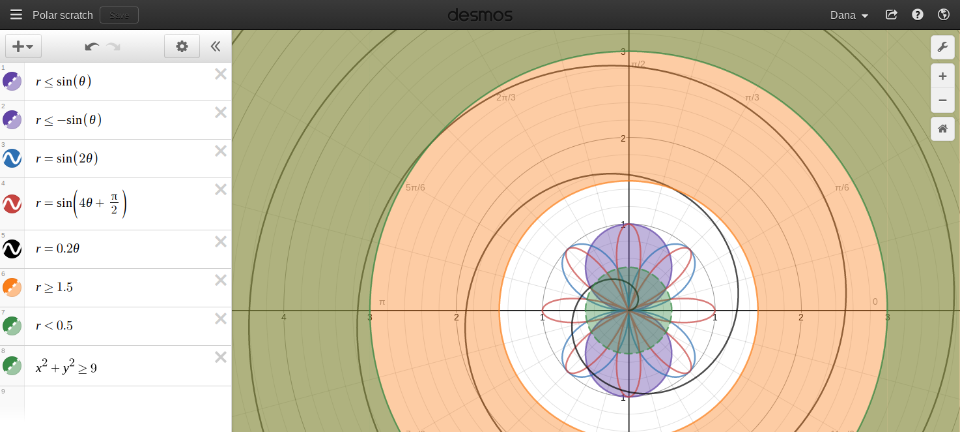Polar art handout

Notes from first run with Desmos. Students quickly developed a formula with sliders for a and b that yield a variety of shapes:
$r=sin\left(\frac{a}{b}\theta \right)$

## Projectile motion

Using a radar gun, I timed a foursquare kickball in flight on low and high arcs. I neglected to attempt to measure the angle of launch, that would be a good addition. I then derived the equation for a projectile launched from y = 0. Homework was built around the equation. After HW review of 7.3, this takes the rest of the period.

## 10.8

### Vectors

Summer 2015 I started class on the sidewalk with a RipStik exercise, using the radar gun to obtain the perpendicular launch velocity of the ball. Details blogged.

Desmos cannot directly handle vector notation.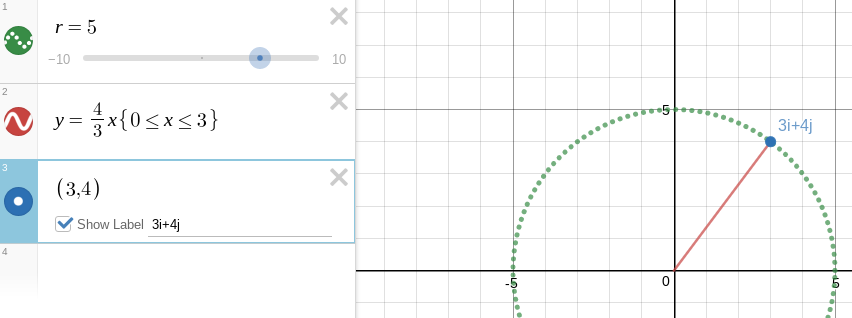Note that one can mix and match the polar variable r and an xy coordinate grid.

Apparently regular parentheses also work. Introduced i, j notation, connection to xy plane. Magnitude is radius is hypotenuse. Angle is arctan(j coefficient/i coefficient).

Scalar multiplication: multiplying the magnitude.

Vector addition: adding vectors. Easiest in i, j form: add the components. If given magnitude and direction angle θ, use r sin θ and r cos θ to find the i, j components. Why vectors add... throwing a ball from a RipStik. Sailing a sail boat. Piloting a ship in a current. Flying a plane in winds aloft. Forces acting on objects. Tension in support lines and guy wires.

After a very brief introduction of magnitude and direction angle, I moved on day two into dot and cross products. Using the physics definitions of the dot product being F d cos(θ) and the cross product being F d sin(θ) where F is the force, d is either the distance the object moves or the lever arm length, and θ is the angle between the two vectors. I used the toy pull cart wagon in my office to illustrate the effect of pulling the cart versus applying a torque and thus lifting the front wheels of the toy wagon up.

I wanted the students to see some mathematical operators beyond what they had seen thus far in life, to lift them higher up on the mountain of functions.

This also allowed me to introduce a reason why I use asterisk for multiplication, besides its default use spreadsheets, programming languages, and WolframAlpha this way. I save dots and crosses for dot and cross products. Larson covers dot products but in a horribly obtuse and obscure fashion with the throttle set to full theoretic. Then, as always, "real world" problems are glued on during the homework.

For the problems below, calculate the work done using the dot product, calculate the torque using the cross product.

## 9.1

### Equations in multiple unknowns

solve x+y=4,x-y=2
solve 3 x^2+4 y=4,2 x-y=-1

The red solution dot on the graph can be hovered to reveal the decimal answer.

SectionEditionPagesProblem set
9.1766649, 52, 53, 55, 70*
866255, 56, 57, 59, 74*

*See the note on plotting multiple functions and the need to omit the dependent variable.

## shapes

A sphere at the origin, radius 5: Sphere[{0, 0, 0}, 5]
Sphere, defined mathematically: Sphere
Sphere, defined mathematically: Sphere
Cylinder, defined mathematically: cylinder
Platonic solids: Platonic solids
Archimedean solids: Archimedean solids